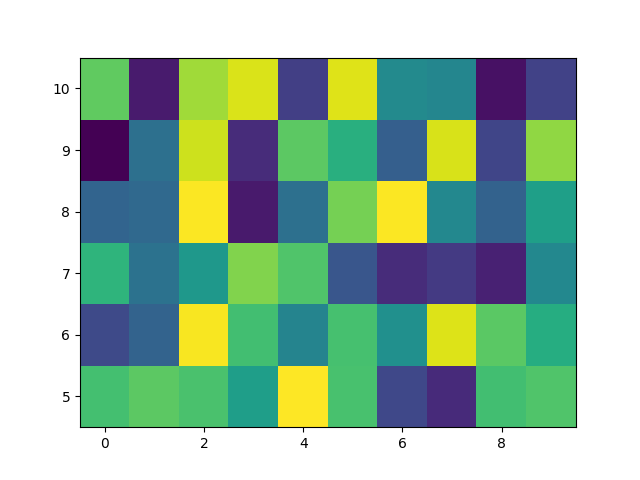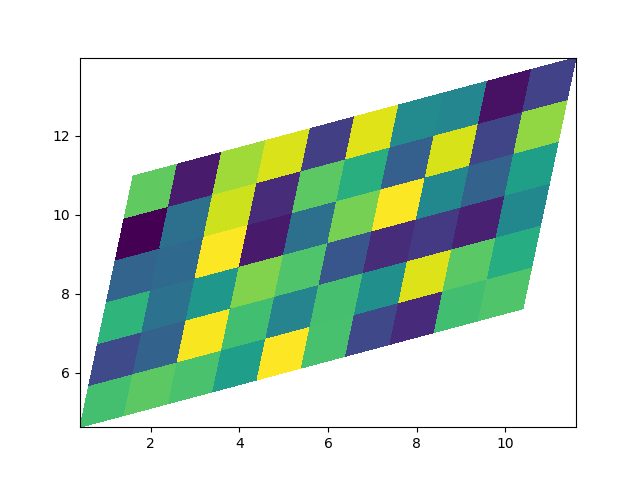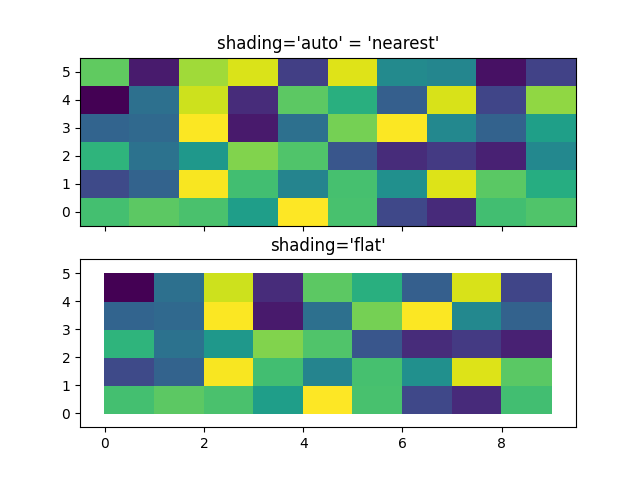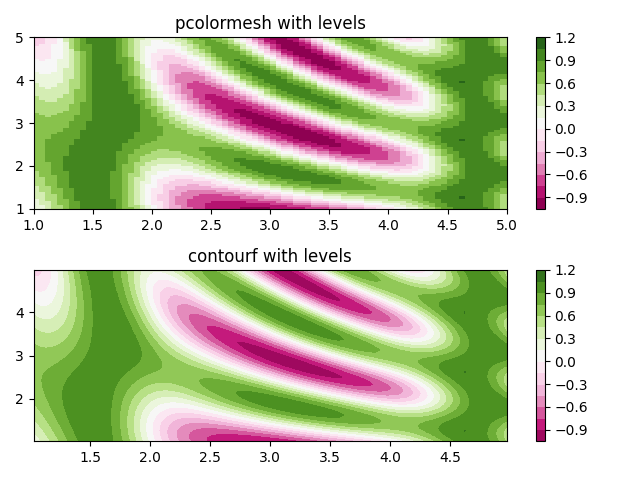# pcolormesh¶

axes.Axes.pcolormesh allows you to generate 2-D image-style plots. Note it is faster than the similar pcolor.

import matplotlib
import matplotlib.pyplot as plt
from matplotlib.colors import BoundaryNorm
from matplotlib.ticker import MaxNLocator
import numpy as np


## Basic pcolormesh¶

We usually specify a pcolormesh by defining the edge of quadrilaterals and the value of the quadrilateral. Note that here x and y each have one extra element than Z in the respective dimension.

np.random.seed(19680801)
Z = np.random.rand(6, 10)
x = np.arange(-0.5, 10, 1)  # len = 11
y = np.arange(4.5, 11, 1)  # len = 7

fig, ax = plt.subplots()
ax.pcolormesh(x, y, Z)Out:

<matplotlib.collections.QuadMesh object at 0x7fcbe282cd30>


## Non-rectilinear pcolormesh¶

Note that we can also specify matrices for X and Y and have non-rectilinear quadrilaterals.

x = np.arange(-0.5, 10, 1)  # len = 11
y = np.arange(4.5, 11, 1)  # len = 7
X, Y = np.meshgrid(x, y)
X = X + 0.2 * Y  # tilt the coordinates.
Y = Y + 0.3 * X

fig, ax = plt.subplots()
ax.pcolormesh(X, Y, Z)Out:

<matplotlib.collections.QuadMesh object at 0x7fcc1125c2b0>


## Centered Coordinates¶

Often a user wants to pass X and Y with the same sizes as Z to axes.Axes.pcolormesh. This is also allowed if shading='auto' is passed (default set by rcParams["pcolor.shading"] (default: 'flat')). Pre Matplotlib 3.3, shading='flat' would drop the last column and row of Z; while that is still allowed for back compatibility purposes, a DeprecationWarning is raised.

x = np.arange(10)  # len = 10
y = np.arange(6)  # len = 6
X, Y = np.meshgrid(x, y)

fig, axs = plt.subplots(2, 1, sharex=True, sharey=True)
axs.pcolormesh(X, Y, Z, vmin=np.min(Z), vmax=np.max(Z), shading='auto')
axs.pcolormesh(X, Y, Z, vmin=np.min(Z), vmax=np.max(Z), shading='flat')Out:

/root/matplotlib/examples/images_contours_and_fields/pcolormesh_levels.py:67: MatplotlibDeprecationWarning: shading='flat' when X and Y have the same dimensions as C is deprecated since 3.3.  Either specify the corners of the quadrilaterals with X and Y, or pass shading='auto', 'nearest' or 'gouraud', or set rcParams['pcolor.shading'].  This will become an error two minor releases later.
axs.pcolormesh(X, Y, Z, vmin=np.min(Z), vmax=np.max(Z), shading='flat')



## Making levels using Norms¶

Shows how to combine Normalization and Colormap instances to draw "levels" in axes.Axes.pcolor, axes.Axes.pcolormesh and axes.Axes.imshow type plots in a similar way to the levels keyword argument to contour/contourf.

# make these smaller to increase the resolution
dx, dy = 0.05, 0.05

# generate 2 2d grids for the x & y bounds
y, x = np.mgrid[slice(1, 5 + dy, dy),
slice(1, 5 + dx, dx)]

z = np.sin(x)**10 + np.cos(10 + y*x) * np.cos(x)

# x and y are bounds, so z should be the value *inside* those bounds.
# Therefore, remove the last value from the z array.
z = z[:-1, :-1]
levels = MaxNLocator(nbins=15).tick_values(z.min(), z.max())

# pick the desired colormap, sensible levels, and define a normalization
# instance which takes data values and translates those into levels.
cmap = plt.get_cmap('PiYG')
norm = BoundaryNorm(levels, ncolors=cmap.N, clip=True)

fig, (ax0, ax1) = plt.subplots(nrows=2)

im = ax0.pcolormesh(x, y, z, cmap=cmap, norm=norm)
fig.colorbar(im, ax=ax0)
ax0.set_title('pcolormesh with levels')

# contours are *point* based plots, so convert our bound into point
# centers
cf = ax1.contourf(x[:-1, :-1] + dx/2.,
y[:-1, :-1] + dy/2., z, levels=levels,
cmap=cmap)
fig.colorbar(cf, ax=ax1)
ax1.set_title('contourf with levels')

# adjust spacing between subplots so ax1 title and ax0 tick labels
# don't overlap
fig.tight_layout()

plt.show()